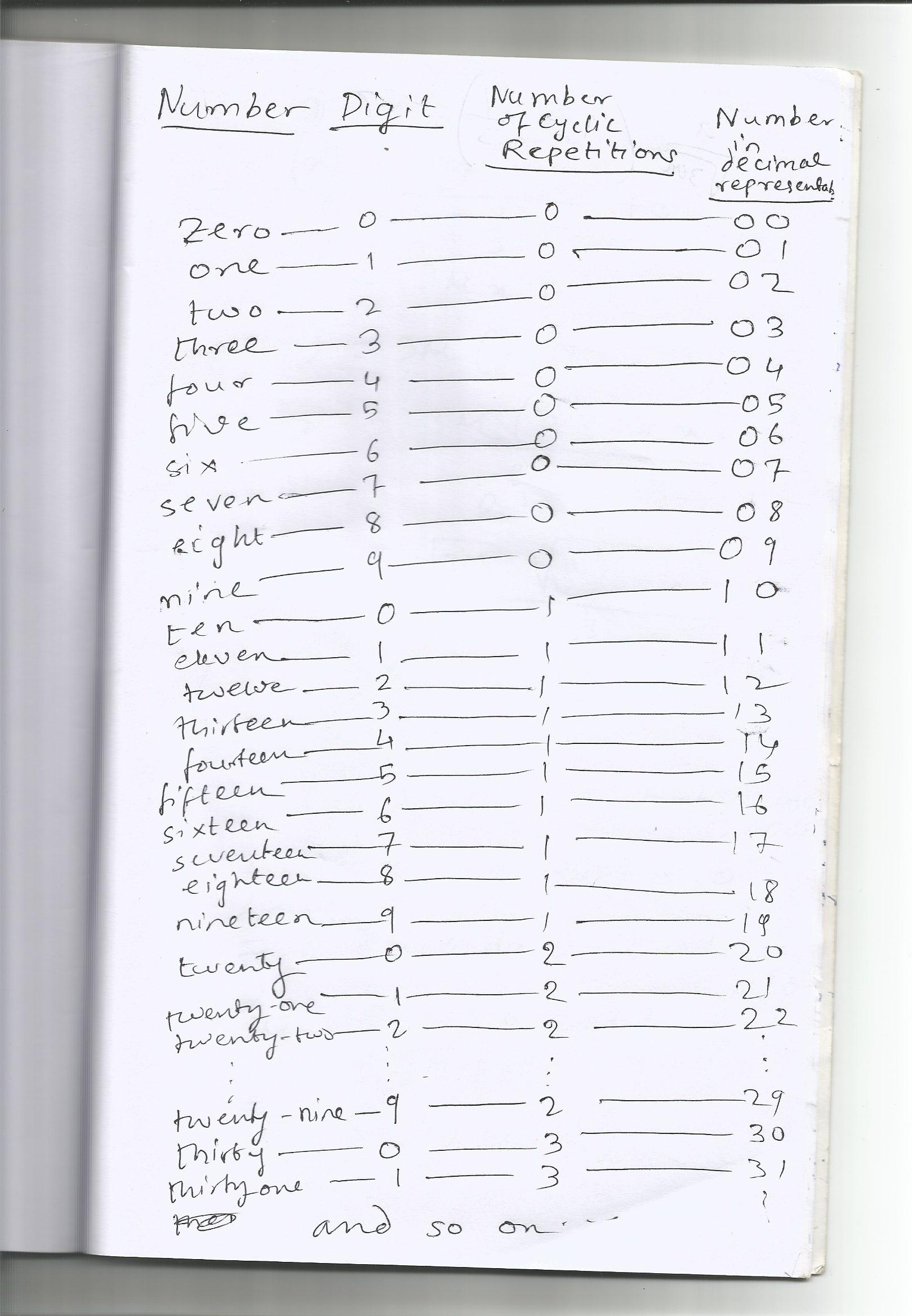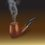# An Explanation for the Decimal System Problem

This is my simple explanation: The decimal system, first proposed by the Vedic Hindus, and then popularized by the Arabians is a positional number system (a one where position of a digit in the number carries some "weight"), and consists of ten fundamental symbols, viz.,0,1,2,3,4,5,6,7,8 and 9. So it is obvious that any number in this system has to be expressed using a combination of these ten digits. Suppose one is independently formulating the decimal system in his or her mind. He or she starts to count: ONE(1), TWO(2), THREE(3), FOUR(4), FIVE(5), SIX(7), SEVEN(7), EIGHT(8), NINE(9)..and then he or she gets stuck with the problem-How to express TEN? There is no digit in the system to express the number TEN. So, the person tries to exploit whatever is in his or her hand - the ten digits. He or she then goes back to the digit 0. But 0 has been already used once in order to express the number ZERO. So in order to express the number TEN, one is taking help of this zero once more. To signify this repetition of usage of the digit 0, he or she places a 1 (that is the number of times 0 is being repeated) to the left of zero. Now the writing of 1 to the left of 0 may be attributed to the fact that the Arabians used to write from right to left, a fact which I am not very much sure of. Thus, what are we doing in this process of constructing the numbers with the help of the ten digits? After the ten digits of the decimal system get exhausted as we denote the first ten whole numbers with them, starting from zero, we come back to 0 again in a cycle to express the numbers from ten onward, writing the number of repetitions on the left of the digit we are using for the particular number. The following image clarifies the explanation:Note by Kuldeep Guha Mazumder
6 years ago

This discussion board is a place to discuss our Daily Challenges and the math and science related to those challenges. Explanations are more than just a solution — they should explain the steps and thinking strategies that you used to obtain the solution. Comments should further the discussion of math and science.

When posting on Brilliant:

• Use the emojis to react to an explanation, whether you're congratulating a job well done , or just really confused .
• Ask specific questions about the challenge or the steps in somebody's explanation. Well-posed questions can add a lot to the discussion, but posting "I don't understand!" doesn't help anyone.
• Try to contribute something new to the discussion, whether it is an extension, generalization or other idea related to the challenge.

MarkdownAppears as
*italics* or _italics_ italics
**bold** or __bold__ bold
- bulleted- list
• bulleted
• list
1. numbered2. list
1. numbered
2. list
Note: you must add a full line of space before and after lists for them to show up correctly
paragraph 1paragraph 2

paragraph 1

paragraph 2

[example link](https://brilliant.org)example link
> This is a quote
This is a quote
    # I indented these lines
# 4 spaces, and now they show
# up as a code block.

print "hello world"
# I indented these lines
# 4 spaces, and now they show
# up as a code block.

print "hello world"
MathAppears as
Remember to wrap math in $$ ... $$ or $ ... $ to ensure proper formatting.
2 \times 3 $2 \times 3$
2^{34} $2^{34}$
a_{i-1} $a_{i-1}$
\frac{2}{3} $\frac{2}{3}$
\sqrt{2} $\sqrt{2}$
\sum_{i=1}^3 $\sum_{i=1}^3$
\sin \theta $\sin \theta$
\boxed{123} $\boxed{123}$